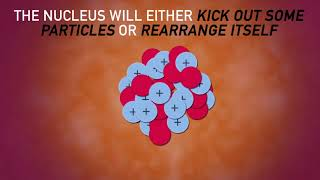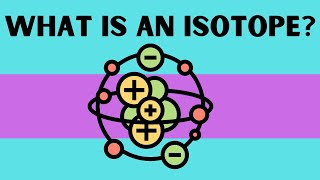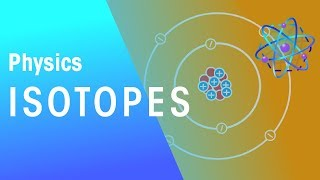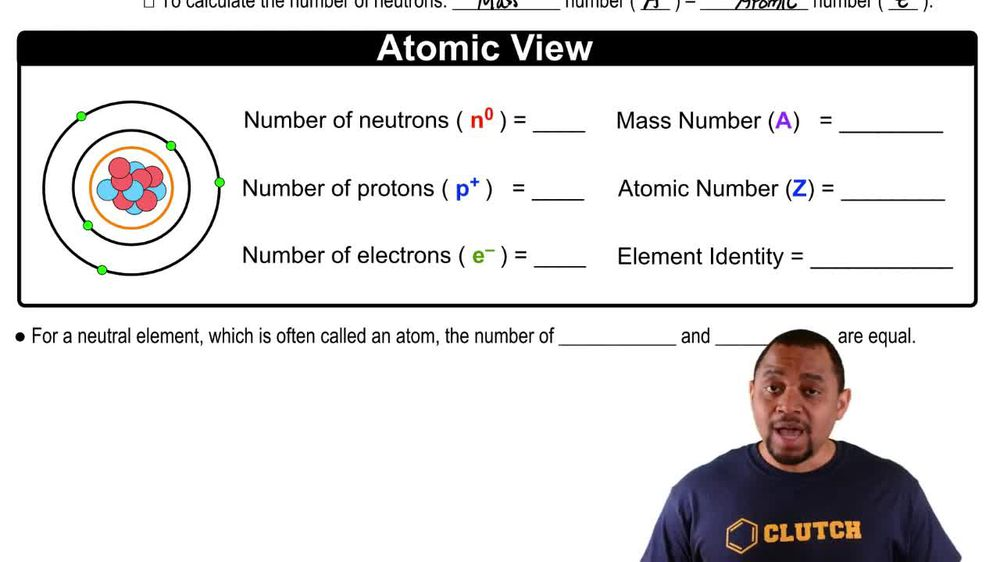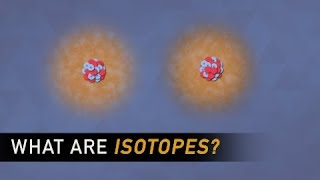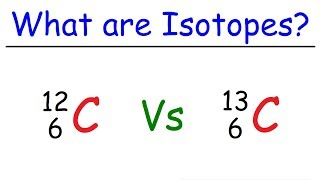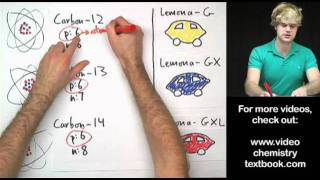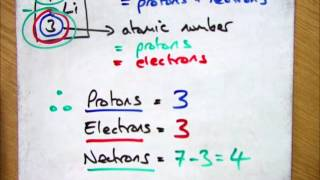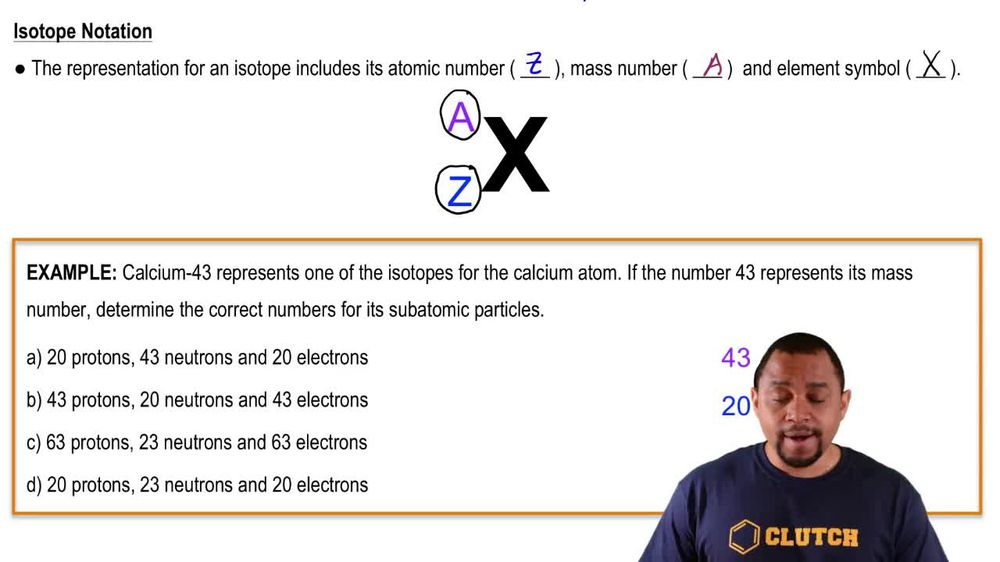Start typing, then use the up and down arrows to select an option from the list.
1. 2. Atoms & Elements2. Isotopes
Problem

# The first atoms of seaborgium (Sg) were identified in 1974. The longest-lived isotope of Sg has a mass number of 266. (a) How many protons, electrons, and neutrons are in an 266Sg atom?

Relevant Solution2m
Play a video:
welcome back everyone in this example, we need to calculate our number of protons neutrons and electrons in our atom of boron 10. So we should recall that in our given symbol for boron. We have this number in the left hand exponents. We should recognize that this number is our mass number or atomic mass. And recall that this is calculated by taking our number of protons and adding that to our number of neutrons for our atom. Now we should also recognize that in our symbol for boron, we're missing our atomic number and we should recall that our atomic number is represented by the symbol Z. And for boron on our product cable we would say that C is equal to a value of five. And so that means that we can fill in our symbol here this value five which corresponds to again our atomic number and recall that this tells us our number of protons and for a neutral atom are number of electrons. And so that means that we would say that therefore we have five protons and five electrons because we have a neutral atom. So right now we've already identified two of our three answered answers. We've identified that we have five protons and five electrons. Now what's left to figure out is how many neutrons we have. And if we know our mass number and we know our number of protons, we just have to subtract the difference to get our number of neutrons. So we would say that our number of neutrons is equal to our mass number of 10 subtracted from our number of protons, which we calculated is five protons. And so that would give us our number of neutrons equal to a value of just five. And so that would be our third and final answer. So we've identified our three answers. We have five protons, five electrons and five neutrons making up our atoms of Boron 10. So I hope that everything I explained was clear what's highlighted in yellow represents our final answers. If you have any questions, just leave them down below and I will see everyone in the next practice video.